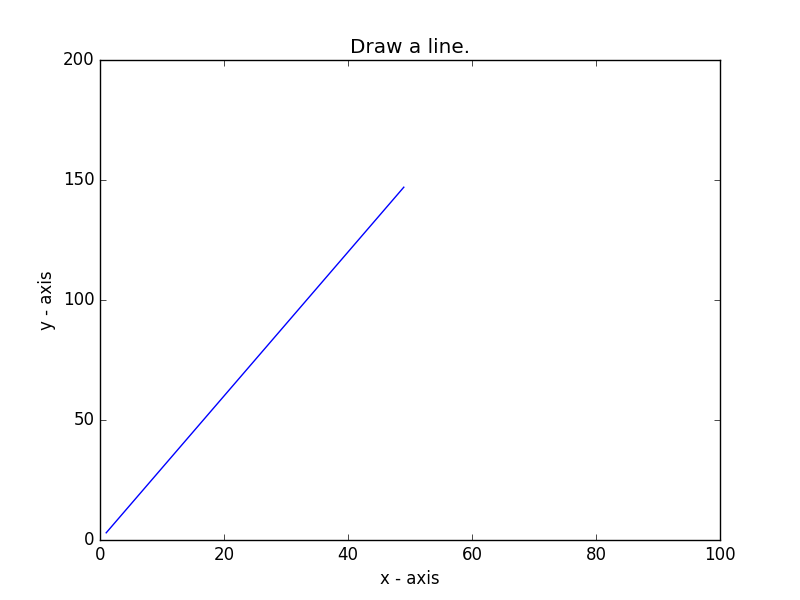﻿ Matplotlib Basic: Display the current axis limits values and set new axis values - w3resource# Matplotlib Basic: Display the current axis limits values and set new axis values

## Matplotlib Basic: Exercise-9 with Solution

Write a Python program to display the current axis limits values and set new axis values.

Sample Solution:

Python Code:

``````import matplotlib.pyplot as plt
X = range(1, 50)
Y = [value * 3 for value in X]
plt.plot(X, Y)
plt.xlabel('x - axis')
plt.ylabel('y - axis')
plt.title('Draw a line.')
# shows the current axis limits values
print(plt.axis())
# set new axes limits
# Limit of x axis 0 to 100
# Limit of y axis 0 to 200
plt.axis([0, 100, 0, 200])
# Display the figure.
plt.show()
```
```

Sample Output:Python Code Editor: